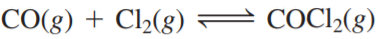×
Get Full Access to Chemistry: A Molecular Approach - 3 Edition - Chapter 14 - Problem 63e
Get Full Access to Chemistry: A Molecular Approach - 3 Edition - Chapter 14 - Problem 63e

×

# Solution: Consider this reaction at equilibrium: PredictISBN: 9780321809247 1

## Solution for problem 63E Chapter 14

Chemistry: A Molecular Approach | 3rd Edition

• Textbook Solutions
• 2901 Step-by-step solutions solved by professors and subject experts
• Get 24/7 help from StudySoup virtual teaching assistantsChemistry: A Molecular Approach | 3rd Edition

4 5 1 275 Reviews
15
4
Problem 63E

Consider this reaction at equilibrium:Predict whether the reaction will shift left, shift right, or remain unchanged after each disturbance:

a. COCl2 is added to the reaction mixture.

b. Cl2 is added to the reaction mixture.

c. COCl2 is removed from the reaction mixture.

Step-by-Step Solution:

Solution 63EStep 1The given chemical reaction:a)When COCI is a2ed to the reaction mixture.According to the Le chatelier’s principle When a chemical system at equilibrium is disturbedthen system shifts in a direction that minimizes the disturbance.When added the COCl reacti2 will shift to left. Because it is a product.Before addition of COCI Q = 2But the moment COCI2 is added i.e. Q > K. the reaction shifts to left.

Step 2 of 3

Step 3 of 3

##### ISBN: 9780321809247

This full solution covers the following key subjects: reaction, mixture, shift, coci, added. This expansive textbook survival guide covers 82 chapters, and 9454 solutions. The full step-by-step solution to problem: 63E from chapter: 14 was answered by , our top Chemistry solution expert on 02/22/17, 04:35PM. Chemistry: A Molecular Approach was written by and is associated to the ISBN: 9780321809247. Since the solution to 63E from 14 chapter was answered, more than 432 students have viewed the full step-by-step answer. This textbook survival guide was created for the textbook: Chemistry: A Molecular Approach, edition: 3. The answer to “?Consider this reaction at equilibrium: Predict whether the reaction will shift left, shift right, or remain unchanged after each disturbance: a. COCl2 is added to the reaction mixture. b. Cl2 is added to the reaction mixture. c. COCl2 is removed from the reaction mixture.” is broken down into a number of easy to follow steps, and 44 words.

## Discover and learn what students are asking

Calculus: Early Transcendental Functions : The Natural Logarithmic Function: Integration
?In Exercises 1-26, find the indefinite integral. $$\int \frac{x}{x^{2}-3} d x$$

Statistics: Informed Decisions Using Data : Scatter Diagrams and Correlation
?A _______ variable is a variable that is related to both the explanatory and response variable.

Chemistry: The Central Science : The Chemistry of Life: Organic and Biological Chemistry
?Identify the functional groups in each of the following compounds:

Unlock Textbook Solution